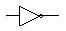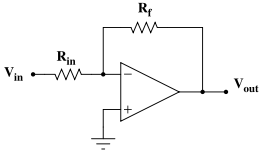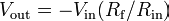Home > Electronic Tutorials > Electronic Schematic Components Symbols > What is Amplifier Inverting / Inverter?

Electronics Component Symbols

# What is Amplifier Inverting / Inverter? ()

The following circuit amplifies a voltage by a factor (R1 / R2).  What is important in this circuit is that it amplifies by almost  exactly (-R1 /R2) so that the gain of the circuit can be controlled precisely by controlling the resistor values precisely.

Inverting amplifierInverts and amplifies a voltage (multiplies by a negative constant)$V_\mathrm{out} = -V_\mathrm{in} ( R_\mathrm{f} / R_\mathrm{in} ) \!\$
• Zin = Rin (because V is a virtual ground)
• A third resistor, of value$R_\mathrm{f} \| R_\mathrm{in} = R_\mathrm{f} R_\mathrm{in} / (R_\mathrm{f} + R_\mathrm{in})$, added between the non-inverting input and ground, while not necessary, minimizes errors due to input bias currents.
Note: To report broken links or to submit your projects please send email to Webmaster

 Discover